Transfer function third order T-CLC low pass - discrepancies

nfh

Joined Jul 6, 2019
10
Hello,

I'm just comparing two transfer function for a simple T-CLC low pass with load and inner resistance of the voltage source taken care of.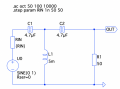One transfer function is obtained numerically via LT-Spice and looks like I expect (blue RIN=50Ω, black RIN->0Ω):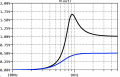However if I calculate the transfer function analytically I seem to mess up. It looks qualitatively fine, but neither maximal amplitude nor cut-off frequency fit (blue RIN=50Ω, black RIN->0Ω):I tried to obtain the transfer function via input and output impedance as
$|\mathcal{H}(i \omega)| = | \frac{Z_a}{Z_e}| \\ Z_a =\frac{R_1 (R_{in}+i L \omega )}{R_1+(R_{in}+i L \omega )} \\ Z_e =\frac{2}{i C \omega }+R_{in}+Z_a$

both capacities shall be identical.

Where is my mistake?

The Electrician

Joined Oct 9, 2007
2,866
Your mistake is that your method of obtaining the transfer function didn't get the correct result.

In order to help you find your mistake you must explain how you got Za and Ze, and why you believe that their ratio gives you the transfer function.

nfh

Joined Jul 6, 2019
10
$$Z_a$$ is my output impedance where my output voltage drops of; that's a parallel circuit of $$L \| R_1$$. $$Z_e$$ is my input impedance, i.e. the inner Resistance $$R_{in}$$ in series to both identical capacitors plus the output impedance. Then it is obviously (?!) $|\mathcal{H}(i\omega)|=|\frac{U_a}{U_e}|=|\frac{Z_a I_a}{Z_e I_e}|=|\frac{Z_a}{Z_e}|$.

LvW

Joined Jun 13, 2013
1,360
$$Z_a$$ is my output impedance where my output voltage drops of; that's a parallel circuit of $$L \| R_1$$. $$Z_e$$ is my input impedance, i.e. the inner Resistance $$R_{in}$$ in series to both identical capacitors plus the output impedance. Then it is obviously (?!) $|\mathcal{H}(i\omega)|=|\frac{U_a}{U_e}|=|\frac{Z_a I_a}{Z_e I_e}|=|\frac{Z_a}{Z_e}|$.
Obviously? Please, can you explain? Is this ratio identical to the classical definition for a voltage transfer function?

nfh

Joined Jul 6, 2019
10
Obviously? Please, can you explain? Is this ratio identical to the classical definition for a voltage transfer function?
That's why I put (?!) behind the obviously. I'm not sure about it. The definition is surely $|\mathcal{H}(i\omega)|=|\frac{U_a}{U_e}|$ so I usually translate it to the impedances as $|\frac{Z_a I_a}{Z_e I_e}|=|\frac{Z_a}{Z_e}|$ with the restriction that incoming and outgoing currents are identical. Which should be.

That method worked for several first and second order passes for me. Here it fails.

MrAl

Joined Jun 17, 2014
8,612
Hello,

I'm just comparing two transfer function for a simple T-CLC low pass with load and inner resistance of the voltage source taken care of.

View attachment 252286

One transfer function is obtained numerically via LT-Spice and looks like I expect (blue RIN=50Ω, black RIN->0Ω):

View attachment 252287

However if I calculate the transfer function analytically I seem to mess up. It looks qualitatively fine, but neither maximal amplitude nor cut-off frequency fit (blue RIN=50Ω, black RIN->0Ω):

View attachment 252288

I tried to obtain the transfer function via input and output impedance as
$|\mathcal{H}(i \omega)| = | \frac{Z_a}{Z_e}| \\ Z_a =\frac{R_1 (R_{in}+i L \omega )}{R_1+(R_{in}+i L \omega )} \\ Z_e =\frac{2}{i C \omega }+R_{in}+Z_a$

both capacities shall be identical.

Where is my mistake?
Hello,

How do you calculate output impedance?
Also, how do you calculate the input impedance?
There are tests we can do to make sure they are both correct.

Also, how do you get an output impedance that does not include any capacitance?
Also, where do you get the formula for the transfer function from the input/output impedances?

If you calculate the input and output impedance for a simple RC series circuit then make the division Zo/Zi you do not get the transfer function you get a second order transfer function.

Also, strictly speaking, the norm of any impedance or impedances can not be the transfer function, however it can be the amplitude. The transfer function is not the same as the amplitude of the output, again strictly speaking.

I think a good idea would be to show your work on a voltage divider made up of two resistors.
The input a voltage source and the output taken across one resistor.
That will clearly show what you are doing and if you are making any mistakes it will be clear i think.

Last edited:
•nfh

nfh

Joined Jul 6, 2019
10
How do you calculate output impedance?
Also, how do you calculate the input impedance?
There are tests we can do to make sure they are both correct.
Also, where do you get the formula for the transfer function from the input/output impedances?

That's explained in the first posts

Also, how do you get an output impedance that does not include any capacitance?

The output voltage is only across an inductor and the load resistor.

If you calculate the input and output impedance for a simple RC series circuit then make the division Zo/Zi you do not get the transfer function you get a second order transfer function.

I do not get that comment. What's the difference?

Also, strictly speaking, the norm of any impedance or impedances can not be the transfer function, however it can be the amplitude. The transfer function is not the same as the amplitude of the output, again strictly speaking.

That's correct. My apologies; that's translational error of mine. The transfer function is meant to be $\mathcal{H}(i\omega)$ while the "amplitude response" is $\mathcal{H}(\omega)= | \mathcal{H}(i\omega)|$.

I think a good idea would be to show your work on a voltage divider made up of two resistors.
The input a voltage source and the output taken across one resistor.
That will clearly show what you are doing and if you are making any mistakes it will be clear i think.

I'll do it for a slightly more complex example: LC-lowpass with load

The voltage at the output is the voltage across the parallel load resistor and the impedance of the capacitor, hence:
$U_a = Z_a I_a = \frac{R_L \cdot \frac{1}{i \omega C}}{R_L + \frac{1}{i \omega C}} I_a$

The voltage generated by the input also has to drop off over the inductor in series, hence it is:
$U_e = Z_e I_e = (i \omega L + Z_a) I_e$

It is$I_a = I_e$

Hence for the transfer function

$\mathcal{H}(i \omega) = \frac{U_a}{U_e} = \frac{R_L \cdot \frac{1}{i \omega C}}{R_L + \frac{1}{i \omega C}} / (i \omega L + Z_a) = \frac{R_L}{-C L R_L \omega^2 + i L \omega + R_L}$

One nicely see's the second order of the pass. If I want to get the amplitude response, I just calculate the absolute values, via imaginary and real parts of the the transfer function. Here in this case I get the following amplitude response: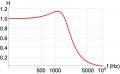Which is perfectly in agreement to my LTSpice simulation: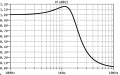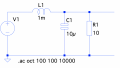It's the same procedure I tried for the third order pass in my starting post. Here (and for others) it works; for the third order one it fails.

MrAl

Joined Jun 17, 2014
8,612
How do you calculate output impedance?
Also, how do you calculate the input impedance?
There are tests we can do to make sure they are both correct.
Also, where do you get the formula for the transfer function from the input/output impedances?

That's explained in the first posts

Also, how do you get an output impedance that does not include any capacitance?

The output voltage is only across an inductor and the load resistor.

If you calculate the input and output impedance for a simple RC series circuit then make the division Zo/Zi you do not get the transfer function you get a second order transfer function.

I do not get that comment. What's the difference?

Also, strictly speaking, the norm of any impedance or impedances can not be the transfer function, however it can be the amplitude. The transfer function is not the same as the amplitude of the output, again strictly speaking.

That's correct. My apologies; that's translational error of mine. The transfer function is meant to be $\mathcal{H}(i\omega)$ while the "amplitude response" is $\mathcal{H}(\omega)= | \mathcal{H}(i\omega)|$.

I think a good idea would be to show your work on a voltage divider made up of two resistors.
The input a voltage source and the output taken across one resistor.
That will clearly show what you are doing and if you are making any mistakes it will be clear i think.

I'll do it for a slightly more complex example: LC-lowpass with load

The voltage at the output is the voltage across the parallel load resistor and the impedance of the capacitor, hence:
$U_a = Z_a I_a = \frac{R_L \cdot \frac{1}{i \omega C}}{R_L + \frac{1}{i \omega C}} I_a$

The voltage generated by the input also has to drop off over the inductor in series, hence it is:
$U_e = Z_e I_e = (i \omega L + Z_a) I_e$

It is$I_a = I_e$

Hence for the transfer function

$\mathcal{H}(i \omega) = \frac{U_a}{U_e} = \frac{R_L \cdot \frac{1}{i \omega C}}{R_L + \frac{1}{i \omega C}} / (i \omega L + Z_a) = \frac{R_L}{-C L R_L \omega^2 + i L \omega + R_L}$

One nicely see's the second order of the pass. If I want to get the amplitude response, I just calculate the absolute values, via imaginary and real parts of the the transfer function. Here in this case I get the following amplitude response:

View attachment 252297

Which is perfectly in agreement to my LTSpice simulation:

View attachment 252298

View attachment 252299

It's the same procedure I tried for the third order pass in my starting post. Here (and for others) it works; for the third order one it fails.

Hello again,

Thanks for the reply and some more information, but it always makes me wonder why when people are asked a simple task they want to complicate it. I asked for a simple calculation of input and output impedance of a simple resistive voltage divider. That makes it a very simple circuit to analyze in several different ways. So could you please do a simple R1, R2 voltage divider next.

"I'll do it for a slightly more complex example: LC-lowpass with load"
I have to wonder why you had to specify "with load" because any network that is going to be inserted into any other circuit will always have a load as well as an input source impedance, and the load has to be matched to the output impedance for maximum power transfer, and same with the input impedance matching. What this means is you would have to calculate the input and output impedances simultaneously. It makes sense that if the output isnt yet loaded you cant possibly know what the input impedance is unless the output is current isolated from the input (voltage buffered).
The same goes for the output impedance. You can not calculate the output impedance without having the required input source impedance in place. Usually the input impedance will match the source impedance, and the output impedance will match the load impedance. In this case, i believe Zout/Zin will work.

So there is a slight bit of complexity here that can be cleared up in 10 seconds if we look at a resistive voltage divider, or at the very least show us what is going on here. It should take you about 30 seconds to type that out i would imagine.

What i can then promise is that we will find out definitively what is going onWhat i suspect so far is that there is a misunderstanding of what exactly input and output impedance is. For example if we just tack on any load to a network output then we have simultaneously altered the output impedance unless that load happened to be of the right output impedance, so any calculation that involves the output impedance (and input impedance) is most likely going to be wrong.
This sounds strange but it's actually very simple to understand.

Last edited:

nfh

Joined Jul 6, 2019
10
Hello again,

Thanks for the reply and some more information, but it always makes me wonder why when people are asked a simple task they want to complicate it. I asked for a simple calculation of input and output impedance of a simple resistive voltage divider. That makes it a very simple circuit to analyze in several different ways. So could you please do a simple R1, R2 voltage divider next.

"I'll do it for a slightly more complex example: LC-lowpass with load"
I have to wonder why you had to specify "with load" because any network that is going to be inserted into any other circuit will always have a load as well as an input source impedance, and the load has to be matched to the output impedance for maximum power transfer, and same with the input impedance matching. What this means is you would have to calculate the input and output impedances simultaneously. It makes sense that if the output isnt yet loaded you cant possibly know what the input impedance is unless the output is current isolated from the input (voltage buffered).
The same goes for the output impedance. You can not calculate the output impedance without having the required input source impedance in place. Usually the input impedance will match the source impedance, and the output impedance will match the load impedance. In this case, i believe Zout/Zin will work.

So there is a slight bit of complexity here that can be cleared up in 10 seconds if we look at a resistive voltage divider, or at the very least show us what is going on here. It should take you about 30 seconds to type that out i would imagine.

What i can then promise is that we will find out definitively what is going onHi, people do the more complicated one, as it is closer to their problem and they are somehow narrow-mindedI did the more specific one as it had complex impedances in it instead of pure real resistors. I added a load resistor, as it is in my case. And of course you can calculate a transfer function without any load and/or impedance matching. Honestly that's done most of the time in text books, while it's not realistic.
But first of all, can you tell me what's wrong or right with my second order low pass, why is it working?! There is clearly no impedance matching at a all. The transfer function is correct imho. That's what I'm interested in. Why is the concept not working for the third order. Or can someone tell me (without Laplace transformation) how to gain the transfer function of the first pass.

However, here the same procedure for the voltage divider:

$Z_a = R_2 \\ Z_e = R_1 + R_2 \\ \mathcal{H}(\omega)=|\mathcal{H}(i\omega)| = \frac{U_a}{U_e} = \frac{Z_a I}{Z_e I} = \frac{R_2}{R_1+R_2}$

MrAl

Joined Jun 17, 2014
8,612
Hi, people do the more complicated one, as it is closer to their problem and they are somehow narrow-mindedI did the more specific one as it had complex impedances in it instead of pure real resistors. I added a load resistor, as it is in my case. And of course you can calculate a transfer function without any load and/or impedance matching. Honestly that's done most of the time in text books, while it's not realistic.
But first of all, can you tell me what's wrong or right with my second order low pass, why is it working?! There is clearly no impedance matching at a all. The transfer function is correct imho. That's what I'm interested in. Why is the concept not working for the third order. Or can someone tell me (without Laplace transformation) how to gain the transfer function of the first pass.

However, here the same procedure for the voltage divider:

$Z_a = R_2 \\ Z_e = R_1 + R_2 \\ \mathcal{H}(\omega)=|\mathcal{H}(i\omega)| = \frac{U_a}{U_e} = \frac{Z_a I}{Z_e I} = \frac{R_2}{R_1+R_2}$
Hello again,

Hey thanks for the reply again with the requested information this helps a lot.

Ok i think i see what is happening here. In the voltage divider with R1 and R2 the output impedance is not R2 (i think you are calling R2 the output impedance but correct me if i am wrong).

We can show that R2 is not the output impedance by doing a little experiment. If we calculate the voltage output unloaded we get:
Vout=Vin*R2/(R1+R2)
which is the basic transfer function for voltage on an unloaded network.
Now if we connect a matching network to the output with the impedance that we believe is the output impedance which we think is R2, then we get:
Vout=Vin*(R2/2)/(R1+R2/2) [R2 would be 1/2 of R2 once the R2 load is applied for the new transfer function calculation]
which of course simplifies to:
Vout=(Vin*R2)/(R2+2*R1)
Now if we looked at the original output without a load we would get the original:
Vout=Vin*R2/(R1+R2)
and if we take 1/2 of that we get:
Vout=Vin*R2/(2*R1+2*R2)


Clearly, the calculation of 1/2 of Vout with no load is not the same as the calculation with the output impedance believed to be correct (R2) so something is not right. If we connected the correct output impedance we would have gotten exactly 1/2 of the original output voltage.


If we do the same experiment with the input impedance which we believed was R1+R2 then when we connect that impedance to the input we should seen the input current decrease by a factor of 1/2. We wont see that either (calculation is fairly simple, R1 now becomes R1+R2+R1).

So what can be do here. Well, we know that if we had both the input and output impedances connected we would see 1/2 and 1/2 as above, but that means that we have to compare it to a network that already has Rs (call Rs the real input impedance) and has Rp (call Rp the real output impedance).

So to start, we can define R1 as both R1 and the real input impedance Rs:
Rx1=R1+Rs

and define R2 as both R2 and the real output impedance:
Rx2=R2*Rp/(R2+Rp)
which is the parallel combination of R2 and Rp.

Now we can calculate the true output voltage:
Vout1=Vin*Rx2/(Rx1+Rx2)
and the output voltage with just Rs added (ie no output load yet):
Vout2=Vin*R2/(R2+Rx1)

and we do the same with the input developing the expressions for Iin1 and Iin2.
Now we have two comparisons to work with so we can set up two equation:
Vout1=Vout2/2
Iin1=Iin2/2

and lastly solve for Rs and for Rp simultaneously.
Now when we calculate Rp/Rs we get Vout=Vin*R2/(R1+R2) which is the transfer function.

The only explanation i can think of is that either it was a coincidence that the simpler calculation works, or maybe it works on some networks, or maybe there is another theory involved.

You can try this on your other networks see what you get.

Last edited:
•nfh

The Electrician

Joined Oct 9, 2007
2,866
Hello,

I'm just comparing two transfer function for a simple T-CLC low pass with load and inner resistance of the voltage source taken care of.

View attachment 252286

One transfer function is obtained numerically via LT-Spice and looks like I expect (blue RIN=50Ω, black RIN->0Ω):

View attachment 252287

However if I calculate the transfer function analytically I seem to mess up. It looks qualitatively fine, but neither maximal amplitude nor cut-off frequency fit (blue RIN=50Ω, black RIN->0Ω):

View attachment 252288

I tried to obtain the transfer function via input and output impedance as
$|\mathcal{H}(i \omega)| = | \frac{Z_a}{Z_e}| \\ Z_a =\frac{R_1 (R_{in}+i L \omega )}{R_1+(R_{in}+i L \omega )} \\ Z_e =\frac{2}{i C \omega }+R_{in}+Z_a$

both capacities shall be identical.

Where is my mistake?
You are using the phrase "output impedance" in a non-standard way. The generally understood meaning of "output impedance" is the same as the driving point impedance at the output port. See:

The output impedance of your circuit is the impedance looking back from the terminal you have labeled "OUT". That impedance will depend on whether the voltage source U0 is connected to the input terminal or not, since the source U0 in considered to have an internal impedance of zero ohms. Generally a source might have a non-zero impedance and you have accounted for this by placing Rin in series with U0 (with U0 having zero ohms impedance).

But you have used the phrase "output impedance" for an impedance looking forward from the input, which is not the standard usage.

If you use "input impedance" and "output impedance" to mean what is meant by the driving point impedance at a port (input or output port), then the transfer function cannot be derived just from those impedances; you must also know the transfer impedances and use them in your calculation.

It would help if you would repost your schematic from post #1 with the two currents Ia and Ie clearly indicated on it.

Last edited:
•nfh

nfh

Joined Jul 6, 2019
10
You are using the phrase "output impedance" in a non-standard way. The generally understood meaning of "output impedance" is the same as the driving point impedance at the output port. See:

The output impedance of your circuit is the impedance looking back from the terminal you have labeled "OUT". That impedance will depend on whether the voltage source U0 is connected to the input terminal or not, since the source U0 in considered to have an internal impedance of zero ohms. Generally a source might have a non-zero impedance and you have accounted for the by placing Rin in series with U0 (with U0 having zero ohms impedance).

But you have used the phrase "output impedance" for an impedance looking forward from the input, which is not the standard usage.

If you use "input impedance" and "output impedance" to mean what is meant by the driving point impedance at a port (input or output port), then the transfer function cannot be derived just from those impedances; you must also know the transfer impedances and use them in your calculation.

It would help if you would repost your schematic from post #1 with the two currents Ia and Ie clearly indicated on it.
Thank you & the other repliers before!

Yes, indeed I am misusing the term "output impedance" here. With "output impedance" I refer to the impedance where "the output voltage will be obtained/measured" - that's indeed different from the definition you used here. I am a theoretical physicist, never had these kinds of things during my studies, which leaves me as an autodidact. One with weird definitions it seems.

However I furthermore found my mistake from the first post. The analytical derivation I did was for the following circuit, which differs from the initially shown one: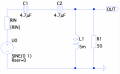Still, has anyone a neat scheme or idea (book recommendations are welcome, too) how to get the transfer function of the first circuit in the opening post without heavy disassembling via KCL?

LvW

Joined Jun 13, 2013
1,360
Still, has anyone a neat scheme or idea (book recommendations are welcome, too) how to get the transfer function of the first circuit in the opening post without heavy disassembling via KCL?
Applying the voltage divider rule for the node between C1 and C2 gives the voltage V1 at this point.
Now you can again apply this rule for the node Vout.

•nfh

The Electrician

Joined Oct 9, 2007
2,866
I would think that a theoretical physicist would like this.Edit: I just realized that these solutions are for the circuit in post #12. See the next post for the solution of the circuit in post #1.

You could use KCL and put the nodal equations in matrix form. To have the simplest possible expressions in every element of the admittance matrix (Y matrix) assign 4 nodes. Let the nodes be: node 1 at the bottom of Rin, node 2 between Rin and C1, node 3 between C1 and C2, node 4 the top of L1 and R1. The first 3 nodes are not essential nodes but they can still be part of an admittance matrix. With these 4 nodes defined, the admittance matrix can be written by inspection, and a solution derived by letting a computer program (Mathematica in this case) do the linear algebra: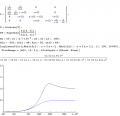Or you could combine Rin, C1, and C2 into one impedance and have a 2x2 matrix instead of 4x4. Now elements of the Y matrix are more complicated, but not unreasonably so: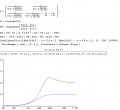Last edited:
•nfh

nfh

Joined Jul 6, 2019
10
Applying the voltage divider rule for the node between C1 and C2 gives the voltage V1 at this point.
Now you can again apply this rule for the node Vout.
I think/thought that's a good idea. I've tried that before, but failed, which the lead me to the first post :/ . Let me show, probably you see a mistake: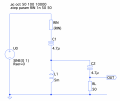$U_{OUT}=\frac{R_L }{R_L+\frac{1}{i \omega C_2}}U_L \\ U_L = \frac{ i L \omega }{\frac{1}{i \omega C_1}+i L \omega + R_{in}} \cdot U_0 \\ \mathcal{H}(i\omega)= \frac{U_{OUT}}{U_0}=\frac{R_L }{R_L+\frac{1}{i \omega C_2}} \cdot \frac{ i L \omega }{\frac{1}{i \omega C_1}+i L \omega + R_{in}}U_0 \\ \mathcal{H}(i\omega) = \frac{C_1 C_2 L R_L \omega ^3}{(C_2 R_L \omega -i) (-1+C_1 \omega (L \omega -i R_{in}))}$

This leads to following frequency response (taking the absolute value and R_in=R_L=50Ohm):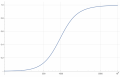That's wrong. I would expect of course only a maximum amplitude ratio of 0.5 ?! Where did I mess up? TY!

Last edited:

nfh

Joined Jul 6, 2019
10
I would think that a theoretical physicist would like this.Edit: I just realized that these solutions are for the circuit in post #12. See the next post for the solution of the circuit in post #1.

You could use KCL and put the nodal equations in matrix form. To have the simplest possible expressions in every element of the admittance matrix (Y matrix) assign 4 nodes. Let the nodes be: node 1 at the bottom of Rin, node 2 between Rin and C1, node 3 between C1 and C2, node 4 the top of L1 and R1. The first 3 nodes are not essential nodes but they can still be part of an admittance matrix. With these 4 nodes defined, the admittance matrix can be written by inspection, and a solution derived by letting a computer program (Mathematica in this case) do the linear algebra:

View attachment 252405

Or you could combine Rin, C1, and C2 into one impedance and have a 2x2 matrix instead of 4x4. Now elements of the Y matrix are more complicated, but not unreasonably so:

View attachment 252402
That's indeed beautiful! You're right at the end it's only linear algebra - my problems seem to be the "... can be written by inspection" - I often not see the forest for the treesThank you!

The Electrician

Joined Oct 9, 2007
2,866
I think/thought that's a good idea. I've tried that before, but failed, which the lead me to the first post :/ . Let me show, probably you see a mistake:

View attachment 252406

$U_{OUT}=\frac{R_L }{R_L+\frac{1}{i \omega C_2}}U_L \\ U_L = \frac{ i L \omega }{\frac{1}{i \omega C_1}+i L \omega + R_{in}} \cdot U_0 \\ \mathcal{H}(i\omega)= \frac{U_{OUT}}{U_0}=\frac{R_L }{R_L+\frac{1}{i \omega C_2}} \cdot \frac{ i L \omega }{\frac{1}{i \omega C_1}+i L \omega + R_{in}}U_0 \\ \mathcal{H}(i\omega) = \frac{C_1 C_2 L R_L \omega ^3}{(C_2 R_L \omega -i) (-1+C_1 \omega (L \omega -i R_{in}))}$

This leads to following frequency response (taking the absolute value and R_in=R_L=50Ohm):

View attachment 252407
That's wrong. I would expect of course only a maximum amplitude ratio of 0.5 ?! Where did I mess up? TY!
The problem is in your equation for UL. The impedance to ground at the top of L1 is not just iLw; the series combination of C2 and RL is in parallel with L1 and you must include their effect.

Last edited:
•nfh

MrAl

Joined Jun 17, 2014
8,612
Thank you & the other repliers before!

Yes, indeed I am misusing the term "output impedance" here. With "output impedance" I refer to the impedance where "the output voltage will be obtained/measured" - that's indeed different from the definition you used here. I am a theoretical physicist, never had these kinds of things during my studies, which leaves me as an autodidact. One with weird definitions it seems.

However I furthermore found my mistake from the first post. The analytical derivation I did was for the following circuit, which differs from the initially shown one:

View attachment 252387

Still, has anyone a neat scheme or idea (book recommendations are welcome, too) how to get the transfer function of the first circuit in the opening post without heavy disassembling via KCL?
Probably what LvW said is the simplest way because many people understand what we sometimes refer to as "The Voltage Divider Rule". This can be applied to various networks that are somewhat simple.
Just in case you have never heard of this, it is very simple. For two resistors in series R1 and R2 and voltage source Vin and taking the output from across R2 we have:
Vout=Vin*R2/(R1+R2)

and that holds to a certain degree for impedances Z1 and Z2 also:
Vout=Vin*Z2/(Z1+Z2)

although i recommend a transient analysis along with any AC analysis or a quick inspection to make sure there are no practical network issues like huge inrush current.

For your original network we could apply the rule twice as i'll illustrate.
First thing is we convert the storage components into their impedances:
zC1=1/(s*C1)
zC2=1/(s*C2)
zL1=s*L1
R1=R1

Now since R1 is in series with C2 we have:
z1=R1+zC2

and since this is in parallel with L1 we have:
z2=zL1*z1/(zL1+z1)

and the impedance in series with the source is:
z3=Rin+zC1

and we end up with the first voltage divider with z3 and z2 taking the output from across z2:
Vx=Vin*z2/(z2+z3)

This si the voltage that appears at the junction of C1, C2, and L1.
To get the output, we can apply the rule again because we know Vx now:
Vout=Vx*R1/(R1+zC2)

and that is the final result which is the transfer function (divide both sides by Vin if you like).

This works with networks where you can combine the component impedances into lumped elements that form one or more voltage dividers.

You will note that in the above we worked from output to input. I like to refer to that as collapsing the network right to left. There is also a left to right procedure also, using Thevenin and/or Norton equivalents. In this technique we collapse the network starting at the input source and convert one source and one impedance at a time until we get to the output and at that point we have the transfer function yet again.
If you'd like to see an illustration i can provide an example.

For myself, i happen to prefer raw Nodal Analysis because it is so general and the procedure is the same for any network we encounter. In both of the above networks we had to assume that the network is easy enough to combine elements but that's not always possible. But dont for a second think i would perform all the math by hand anymore, today we use math software to do the calculations and it is much, much faster, and allows us to do large networks without too much trouble and the math software also takes care of the simultaneous equations that result from the analysis.